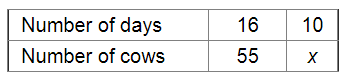# 55 cows can graze a field in 16 days.`
Question:

55 cows can graze a field in 16 days. How many cows will graze the same field in 10 days?

Solution:

Let x be the number of cows that can graze the field in 10 days .Since the number of cows and the number of days taken by them to graze the field are in inverse variation, we have:

$16 \times 55=10 \times x$

$\Rightarrow x=\frac{16 \times 55}{10}$

$=88$

$\therefore$ The required number of cows is 88